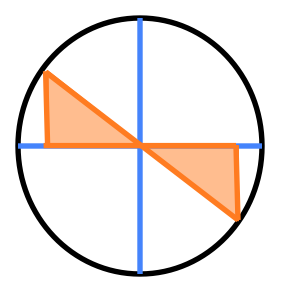### Home > PC3 > Chapter 9 > Lesson 9.1.4 > Problem9-89

9-89.

Solve each of the folling equations, where $0 ≤ x < 2π$.

1. $\csc(x) = −2$

$\frac{1}{\sin(x)}=-2$

1. $\cot(x) = - \frac { \sqrt { 3 } } { 3 }$

What special angle is this?

In what quadrants is tangent negative?